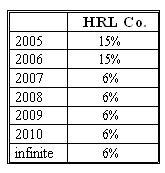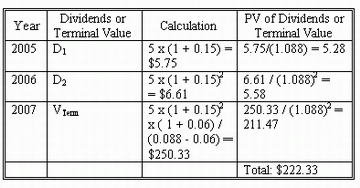### CFA Practice Question

There are 96 practice questions for this study session.

### CFA Practice Question

Beth is evaluating HRL Co. which has been growing at the annual rate of 15% for the last 5 years. Here is her forecast of the company's future dividend growth trend.After considerable study, she has decided to use the following information in her valuation (as of beginning of 2005):

• Beta: 0.8.
• 2004 dividend: \$5.
• The risk-free rate: 4%.

What is the value of the HRL's stock?
A. 211.47.
B. 205.23.
C. 222.33.
Explanation: The company is expected to grow at a constant rate (15%) during the next two years. In year 2007 the growth falls to 6% and stabilizes at this rate. A company with this growth pattern can be valued using the two-stage DDM. HRL's required rate of return is rHRL = rf + beta x market premium = 0.04 + 0.8 x 0.06 = 0.088, or 8.8%.

The following table demonstrates the two-stage DDM valuation of HRL: